Abhishek Sharma — Published On June 30, 2020 and Last Modified On April 26th, 2023

## Introduction

A decision tree is a powerful machine learning algorithm extensively used in the field of data science. They are simple to implement and equally easy to interpret. It also serves as the building block for other widely used and complicated machine-learning algorithms like Random Forest, XGBoost, and LightGBM. I often lean on the decision tree algorithm as my go-to machine learning algorithm, whether I’m starting a new project or competing in a hackathon. In this article, I will explain 4 simple methods for splitting a node in a decision tree.Learning Objectives

• Learn how to split a decision tree based on different splitting criteria.
• Get acquainted with the Reduction in Variance, Gini Impurity, Information Gain, and Chi-square in decision trees.
• Know the difference between these different methods of splitting.

I assume familiarity with the basic concepts in regression and decision trees. Here are two free and popular courses to quickly learn or brush up on the key concepts:

## Basic Decision Tree Terminologies

Let’s quickly go through some of the key terminologies related to decision trees which we’ll be using throughout this article.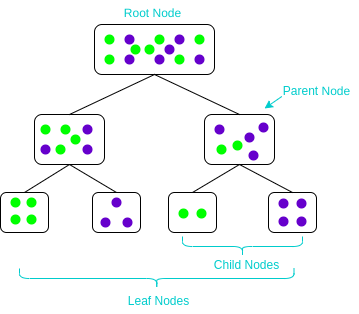• Parent and Child Node: A node that gets divided into sub-nodes is known as Parent Node, and these sub-nodes are known as Child Nodes. Since a node can be divided into multiple sub-nodes, it can act as a parent node of numerous child nodes.
• Root Node: The topmost node of a decision tree. It does not have any parent node. It represents the entire population or sample.
• Leaf / Terminal Nodes: Nodes of the tree that do not have any child node are known as Terminal/Leaf Nodes.

There are multiple tree models to choose from based on their learning technique when building a decision tree, e.g., ID3, CART, Classification and Regression Tree, C4.5, etc. Selecting which decision tree to use is based on the problem statement. For example, for classification problems, we mainly use a classification tree with a gini index to identify class labels for datasets with relatively more number of classes.

## What Is Node Splitting in a Decision Tree & Why Is It Done?

Modern-day programming libraries have made using any machine learning algorithm easy, but this comes at the cost of hidden implementation, which is a must-know for fully understanding an algorithm. Another reason for this infinite struggle is the availability of multiple ways to split decision tree nodes adding to further confusion.

Before learning any topic, I believe it is essential to understand why you’re learning it. That helps in understanding the goal of learning a concept. So let’s understand why to learn about node splitting in decision trees.

Since you all know how extensively decision trees are used, there is no denying the fact that learning about decision trees is a must. A decision tree makes decisions by splitting nodes into sub-nodes. It is a supervised learning algorithm. This process is performed multiple times in a recursive manner during the training process until only homogenous nodes are left. This is why a decision tree performs so well. The process of recursive node splitting into subsets created by each sub-tree can cause overfitting. Therefore, node splitting is a key concept that everyone should know.

Node splitting, or simply splitting, divides a node into multiple sub-nodes to create relatively pure nodes. This is done by finding the best split for a node and can be done in multiple ways. The ways of splitting a node can be broadly divided into two categories based on the type of target variable:

1. Continuous Target Variable: Reduction in Variance
2. Categorical Target Variable: Gini Impurity, Information Gain, and Chi-Square

We’ll look at each splitting method in detail in the upcoming sections. Let’s start with the first method of splitting – reduction in variance.

## Reduction in Variance in Decision Tree

Reduction in Variance is a method for splitting the node used when the target variable is continuous, i.e., regression problems. It is called so because it uses variance as a measure for deciding the feature on which a node is split into child nodes.Variance is used for calculating the homogeneity of a node. If a node is entirely homogeneous, then the variance is zero.

Here are the steps to split a decision tree using the reduction in variance method:

1. For each split, individually calculate the variance of each child node
2. Calculate the variance of each split as the weighted average variance of child nodes
3. Select the split with the lowest variance
4. Perform steps 1-3 until completely homogeneous nodes are achieved

The below video excellently explains the reduction in variance using an example:

## Information Gain in Decision Tree

Now, what if we have a categorical target variable? For categorical variables, a reduction in variation won’t quite cut it. Well, the answer to that is Information Gain. The Information Gain method is used for splitting the nodes when the target variable is categorical. It works on the concept of entropy and is given by: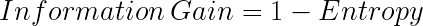Entropy is used for calculating the purity of a node. The lower the value of entropy, the higher the purity of the node. The entropy of a homogeneous node is zero. Since we subtract entropy from 1, the Information Gain is higher for the purer nodes with a maximum value of 1. Now, let’s take a look at the formula for calculating the entropy:Steps to split a decision tree using Information Gain:

1. For each split, individually calculate the entropy of each child node
2. Calculate the entropy of each split as the weighted average entropy of child nodes
3. Select the split with the lowest entropy or highest information gain
4. Until you achieve homogeneous nodes, repeat steps 1-3

Here’s a video on how to use information gain for splitting a decision tree:

## Gini Impurity in Decision Tree

Gini Impurity is a method for splitting the nodes when the target variable is categorical. It is the most popular and easiest way to split a decision tree. The Gini Impurity value is: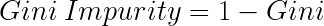Wait – what is Gini?

Gini is the probability of correctly labeling a randomly chosen element if it is randomly labeled according to the distribution of labels in the node. The formula for Gini is: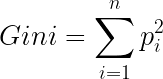And Gini Impurity is: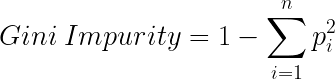The lower the Gini Impurity, the higher the homogeneity of the node. The Gini Impurity of a pure node is zero. Now, you might be thinking we already know about Information Gain then, why do we need Gini Impurity?

Gini Impurity is preferred to Information Gain because it does not contain logarithms which are computationally intensive.

Here are the steps to split a decision tree using Gini Impurity:

1. Similar to what we did in information gain. For each split, individually calculate the Gini Impurity of each child node
2. Calculate the Gini Impurity of each split as the weighted average Gini Impurity of child nodes
3. Select the split with the lowest value of Gini Impurity
4. Until you achieve homogeneous nodes, repeat steps 1-3

And here’s Gini Impurity in video form:

## Chi-Square in Decision Tree

Chi-square is another method of splitting nodes in a decision tree for datasets having categorical target values. It is used to make two or more splits in a node. It works on the statistical significance of differences between the parent node and child nodes.

The Chi-Square value is: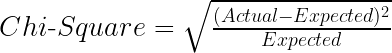Here, the Expected is the expected value for a class in a child node based on the distribution of classes in the parent node, and the Actual is the actual value for a class in a child node.

The above formula gives us the value of Chi-Square for a class. Take the sum of Chi-Square values for all the classes in a node to calculate the Chi-Square for that node. The higher the value, the higher will be the differences between parent and child nodes, i.e., the higher will be the homogeneity.

Here are the steps to split a decision tree using Chi-Square:

1. For each split, individually calculate the Chi-Square value of each child node by taking the sum of Chi-Square values for each class in a node
2. Calculate the Chi-Square value of each split as the sum of Chi-Square values for all the child nodes
3. Select the split with a higher Chi-Square value
4. Until you achieve homogeneous nodes, repeat steps 1-3

Here’s a video explaining Chi-Square in the context of a decision tree:

## Conclusion

Decision trees are an important tool in machine learning for solving classification and regression problems. However, creating an effective decision tree requires choosing the right features and splitting the data in a way that maximizes information gain. After reading the above article, you know about different methods of splitting a decision tree.

Key Takeaways

• We got to learn about splitting in regression and classification problems.
• There is no best method for splitting a decision tree, but there are some methods that are used more than others. It depends on your training data and the problem statement.

In the next steps, you can watch our complete playlist on decision trees on youtube. Or, you can take our free course on decision trees here.

I have also put together a list of fantastic articles on decision trees below:

Q1. What is the best method for splitting a decision tree?

A. The most widely used method for splitting a decision tree is the gini index or the entropy. The default method used in sklearn is the gini index for the decision tree classifier. The scikit learn library provides all the splitting methods for classification and regression trees. You can choose from all the options based on your problem statement and dataset.

Q2. What are the advantages of a decision tree?

A. The main advantage of a decision tree is that it does not require normalization or scaling of data; hence lesser preprocessing is required for data preparation. Moreover, it is easier to understand and interpret as compared to other classification models.

Q3. How many splits are there in a decision tree?

A. For n number of classes in a decision tree, there are 2^(n -1) – 1 maximum splits.###### Abhishek Sharma

He is a data science aficionado, who loves diving into data and generating insights from it. He is always ready for making machines to learn through code and writing technical blogs. His areas of interest include Machine Learning and Natural Language Processing still open for something new and exciting.